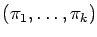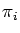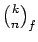Journal of Integer Sequences, Vol. 18 (2015), Article 15.4.1

## Some Elementary Congruences for the Number of Weighted Integer Compositions

### Steffen Eger Computer Science Department Goethe University Frankfurt am Main 60325 Frankfurt am Main Germany

Abstract:

An integer composition of a nonnegative integer n is a tupleof nonnegative integers whose sum is n; the's are called the parts of the composition. For fixed number k of parts, the number of f-weighted integer compositions (also called f-colored integer compositions in the literature), in which each part size s may occur in f(s) different colors, is given by the extended binomial coefficient. We derive several congruence properties for, most of which are analogous to those for ordinary binomial coefficients. Among them is the parity of, Babbage's congruence, Lucas' theorem, etc. We also give congruences for cf(n), the number of f-weighted integer compositions with arbitrarily many parts, and for extended binomial coefficient sums. We close with an application of our results to prime criteria for weighted integer compositions.

Full version:  pdf,    dvi,    ps,    latex

(Concerned with sequences A007318 A027907.)

Received September 15 2014; revised version received February 20 2015. Published in Journal of Integer Sequences, March 25 2015.